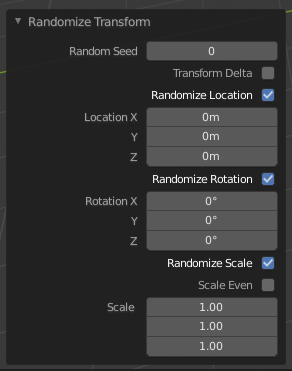# Randomize¶

Reference

Mode

Object Mode and Edit Mode

Object ‣ Transform ‣ Randomize TransformRandomize transform options.

This tool randomizes the move, rotate, and scale values to an object or multiple objects. When applied on multiple objects, each object gets its own seed value, and will get different transform results from the rest.

Random Seed

The random seed is an offset to the randomized transformation. A different seed will produce a new result.

Transform Delta

Randomize Delta Transform values instead of regular transform.

Randomize Location

Randomize Location values.

Location

The maximum distances the objects can move along each axis.

Randomize Rotation

Randomize rotation values.

Rotation

The maximum angle the objects can rotate on each axis.

Randomize Scale

Randomize scale values.

Scale Even

Use the same scale for each axis.

Scale

The maximum scale randomization over each axis.